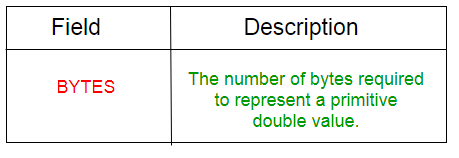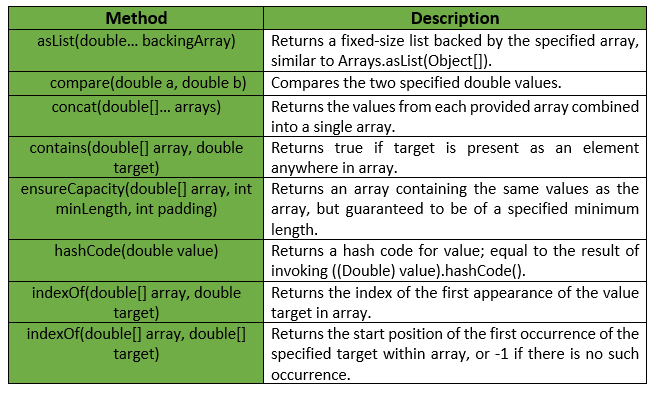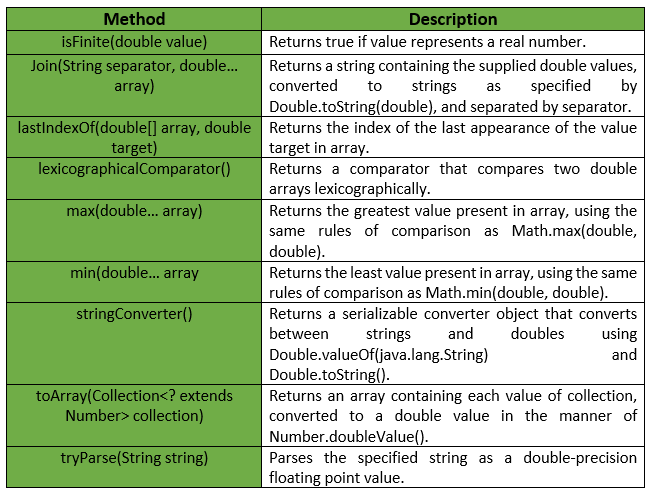# Doubles Class | Guava | Java

Doubles is a utility class for primitive type double. It provides Static utility methods pertaining to double primitives, that are not already found in either Double or Arrays.

Declaration :

```@GwtCompatible(emulated=true)
public final class Doubles
extends Object
```

Below table shows the Field summary for Guava Doubles Class :Some of the methods provided by Guava Doubles Class are :Exceptions :

• min : IllegalArgumentException if array is empty.
• max : IllegalArgumentException if array is empty.
• ensureCapacity : IllegalArgumentException if minLength or padding is negative.
• toArray : NullPointerException if collection or any of its elements is null.

Below table shows some other methods provided by Guava Doubles Class :Below given are some examples showing the implementation of Guava Doubles Class methods :
Example 1 :

 `// Java code to show implementation ` `// of Guava Doubles.asList() method ` ` `  `import` `com.google.common.primitives.Doubles; ` `import` `java.util.*; ` ` `  `class` `GFG { ` `    ``// Driver method ` `    ``public` `static` `void` `main(String[] args) ` `    ``{ ` `        ``double` `arr[] = { ``2.6``, ``4.6``, ``1.2``, ``2.4``, ``1.5` `}; ` ` `  `        ``// Using Doubles.asList() method which ` `        ``// converts array of primitives to array of objects ` `        ``List myList = Doubles.asList(arr); ` ` `  `        ``// Displaying the elements ` `        ``System.out.println(myList); ` `    ``} ` `} `

Output :

```[2.6, 4.6, 1.2, 2.4, 1.5]
```

Example 2 :

 `// Java code to show implementation ` `// of Guava Doubles.toArray() method ` ` `  `import` `com.google.common.primitives.Doubles; ` `import` `java.util.*; ` ` `  `class` `GFG { ` `    ``// Driver method ` `    ``public` `static` `void` `main(String[] args) ` `    ``{ ` `        ``List myList = Arrays.asList(``2.6``, ``4.6``, ``1.2``, ``2.4``, ``1.5``); ` ` `  `        ``// Using Doubles.toArray() method which ` `        ``// converts a List of Doubles to an ` `        ``// array of double ` `        ``double``[] arr = Doubles.toArray(myList); ` ` `  `        ``// Displaying the elements ` `        ``System.out.println(Arrays.toString(arr)); ` `    ``} ` `} `

Output :

```[2.6, 4.6, 1.2, 2.4, 1.5]
```

Example 3 :

 `// Java code to show implementation ` `// of Guava Doubles.concat() method ` ` `  `import` `com.google.common.primitives.Doubles; ` `import` `java.util.*; ` ` `  `class` `GFG { ` `    ``// Driver method ` `    ``public` `static` `void` `main(String[] args) ` `    ``{ ` `        ``double``[] arr1 = { ``2.6``, ``4.6``, ``1.2` `}; ` `        ``double``[] arr2 = { ``2.4``, ``1.5` `}; ` ` `  `        ``// Using Doubles.concat() method which ` `        ``// combines arrays from specified ` `        ``// arrays into a single array ` `        ``double``[] arr = Doubles.concat(arr1, arr2); ` ` `  `        ``// Displaying the elements ` `        ``System.out.println(Arrays.toString(arr)); ` `    ``} ` `} `

Output :

```[2.6, 4.6, 1.2, 2.4, 1.5]
```

Example 4 :

 `// Java code to show implementation ` `// of Guava Doubles.contains() method ` ` `  `import` `com.google.common.primitives.Doubles; ` ` `  `class` `GFG { ` `    ``// Driver method ` `    ``public` `static` `void` `main(String[] args) ` `    ``{ ` `        ``double``[] arr = { ``2.6``, ``4.6``, ``1.2``, ``2.4``, ``1.5` `}; ` ` `  `        ``// Using Doubles.contains() method which ` `        ``// checks if element is present in array ` `        ``// or not ` `        ``System.out.println(Doubles.contains(arr, ``2.5``)); ` `        ``System.out.println(Doubles.contains(arr, ``1.5``)); ` `    ``} ` `} `

output :

```false
true
```

Example 5 :

 `// Java code to show implementation ` `// of Guava Doubles.min() method ` ` `  `import` `com.google.common.primitives.Doubles; ` ` `  `class` `GFG { ` `    ``// Driver method ` `    ``public` `static` `void` `main(String[] args) ` `    ``{ ` `        ``double``[] arr = { ``2.6``, ``4.6``, ``1.2``, ``2.4``, ``1.5` `}; ` ` `  `        ``// Using Doubles.min() method ` `        ``System.out.println(Doubles.min(arr)); ` `    ``} ` `} `

Output :

```1.2
```

Example 6 :

 `// Java code to show implementation ` `// of Guava Doubles.max() method ` ` `  `import` `com.google.common.primitives.Doubles; ` ` `  `class` `GFG { ` `    ``// Driver method ` `    ``public` `static` `void` `main(String[] args) ` `    ``{ ` `        ``double``[] arr = { ``2.6``, ``4.6``, ``1.2``, ``2.4``, ``1.5` `}; ` ` `  `        ``// Using Doubles.max() method ` `        ``System.out.println(Doubles.max(arr)); ` `    ``} ` `} `

Output :

```4.6
```

Attention reader! Don’t stop learning now. Get hold of all the important Java and Collections concepts with the Fundamentals of Java and Java Collections Course at a student-friendly price and become industry ready.

My Personal Notes arrow_drop_upIn love with a semicolon because sometimes i miss it so badly)

If you like GeeksforGeeks and would like to contribute, you can also write an article using contribute.geeksforgeeks.org or mail your article to contribute@geeksforgeeks.org. See your article appearing on the GeeksforGeeks main page and help other Geeks.

Please Improve this article if you find anything incorrect by clicking on the "Improve Article" button below.

Article Tags :
Practice Tags :

Be the First to upvote.

Please write to us at contribute@geeksforgeeks.org to report any issue with the above content.